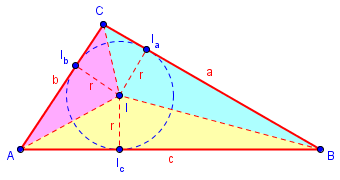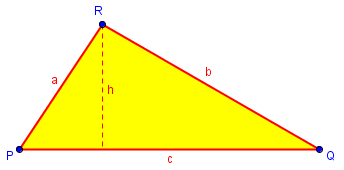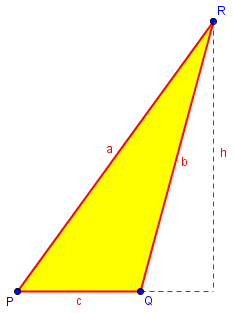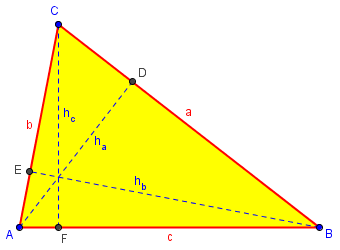# Area of a Triangle: Heron’s Formula II

Last time we looked at a very useful formula for finding the area of any triangle, given only the lengths of its sides. Today I want to look at several problems in which the formula has been used, some of them surprising.

## Area of a plot of land

Calculating Areas of Quadrilaterals and Triangles

What portion of an acre is a plot of land 85 x 174 x 73 x 118?  What portion of an acre is a plot of land 150 x 118 x 150?

I'm not sure of the dimensions of an acre.

Doctor Rick took this:

This is perhaps the most common question we get that we cannot answer! (At least your first question.)

When you want to find the area of a quadrilateral (figure with four straight sides), it isn't enough to know the lengths of the four sides.  If you can imagine taking four sticks with lengths 85, 174, 73, and 118 inches, and hinging them together at the ends, you'll find that the result is not a rigid figure.  You can change the angles between the sides, and the area will change as you do that.

If, in addition to the lengths of the sides, you also knew the angle between two of the sides, we could find the area.  (If you have a site plan that shows not just the lengths but the directions of the sides, we could find the angles between sides.)  Or if you could measure the length of one of the diagonals, the distance between opposite corners of the lot, that would be enough information too.

I’ll soon be posting about finding areas of randomly shaped lots, which as Doctor Rick said is a very common question. Some methods we have taught use angles, and some use diagonal lengths.

Now for the second question: if you're saying that this lot is a triangle, the lengths of the sides *are* sufficient to find the area. If you try the same hinged-stick experiment, you'll find that the triangle is rigid.

The formula to use here is Heron's formula for the area of a triangle:

Area = sqrt(s(s-a)(s-b)(s-c))

where a, b, and c are the sides of the triangle, and

s = (a+b+c)/2

is the "semi-perimeter" (half the perimeter) of the triangle.  With your numbers, we get

a = 150
b = 118
c = 150
s = (150+118+150)/2 = 209
Area = sqrt(209*59*91*59)
= sqrt(66205139)
= 8136.7

If the lengths are in feet, the area of the triangle is 8136.7 square feet.  One acre is 43,560 square feet, so the area is

1 acre
8136.7 ft^2 * ----------- = 0.18679 acre
43,560 ft^2

If you can get a diagonal of the quadrilateral in your first question, you can use this same method to find the area of each of the triangles into which the diagonal divides the lot.  The area of the lot is the sum of these two areas.

We’ll find areas of some more complicated plots at the end of this series.

## Area of a triangle in two ways

Here is an example where the complication is not big, real-life numbers, but radicals:

Heron's Formula, Cartesian Coordinate Plane

If a triangle has sides 5, 6, and the square root of thirteen, what is the area of the triangle?

Doctor Greenie took the opportunity to discuss how to decide what method to use:

Hi, Jacob -

Cool problem! Thanks for sending it to us.

When I am given the lengths of the three sides of a triangle and am asked to find the area, I have the following thoughts:

(1) Maybe the lengths of the sides satisfy the Pythagorean theorem, indicating the triangle is a right triangle; then finding its area is easy.

(2) If the triangle is not a right triangle, then maybe I can draw it on a Cartesian coordinate plane in such a way that its area is easy to determine.

(3) If (1) and (2) don't get me anywhere, there is always Heron's formula:

Area = sqrt[(s)(s-a)(s-b)(s-c)]

where a, b, and c are the lengths of the sides and s is the semi-perimeter, s = (a+b+c)/2


Although Heron’s formula is nice, notice that, because it can involve difficult calculations, it is generally the last one to consider. This is a good general principle: Check for easy ways first, rather than always grabbing the same tool for every problem.

For the particular problem you give....

(1) The given lengths do not satisfy the Pythagorean theorem, so the triangle is not a right triangle.

(2) I looked at this for a while and didn't see anything right away.  So I went on to Heron's formula, with the idea of coming back to look at this approach some more.

(3) The use of Heron's formula looks formidable, with one side having length sqrt(13) - but the arithmetic - thanks to "(a+b)(a-b) = a^2-b^2" - turns out not to be at all bad. Here it is:

With a = 5, b = 6, and c = sqrt(13), we have s = (11+sqrt(13))/2.  Then we have

A = sqrt[(s)(s-a)(s-b)(s-c)]

(11+sqrt(13))   (11-sqrt(13))   sqrt(13)+1   sqrt(13)-1
A = sqrt(------------- * ------------- * ---------- * ----------)
2               2              2            2

121-13   13-1
A = sqrt(------ * ----)
4       4

108*12
A = sqrt(------)
16

A = sqrt(27*3)

A = sqrt(81)

A = 9

Pay attention to the nice way he was able to simplify the radicand by multiplying factors in the easiest order. Again, take the time to look for an easy way before plowing through.

But there is, it turns out, an easier way:

Now let's go back and look at approach (2). I had noticed that the side with length sqrt(13) could be represented by the hypotenuse of a right triangle with sides of length 2 and 3; so I drew a right triangle on a Cartesian coordinate plane with vertices A(0,0), B(2,0), and C(0,3):

|
C
|
*
* *
*   *
*     *
*       *
*         *
*           *
--------------------------*-*-*-*-*-*-*-*-------------
A |               B
|

Then I thought about how I could use BC, with length sqrt(13), as one of the sides of the triangle in the problem. With a little playing around, I discovered that, if I chose point D(-4,0), I would have AD = 4, which with AC = 3 means CD = 5; then DB = DA+AB = 4+2 = 6.  So then triangle BCD is a triangle with sides 5, 6, and sqrt(13), as required:

|
C
|
*
*   * *
*       *   *
*           *     *
*               *       *
*                   *         *
*                       *           *
----*-*-*-*-*-*-*-*-*-*-*-*-*-*-*-*-*-*-*-*-*-*-------------
D                         A |               B
|

The area of this triangle is easy to calculate, because it has a base of 6 and a height of 3: A = one-half base times height = (6*3)/2 = 9.

Thanks again for a nice problem. It is always fun for me to find an unusual problem that has a very neat solution.

Probably this last method was motivated by an expectation that the answer would be simple, so that it would be worth looking for a nice relationship within the triangle. In real life, one would not try this.

## Area of an inscribed circle

The next question, from 1998, deals with the incircle, which we mentioned last time:

Area of Inscribed Circle

The question is: Find the area of the circle inscribed in a triangle a, b, c.  The hint is: Draw lines to the center of the circle and use Heron's law.

Here is a picture, which we saw as part of the third proof last time:Doctor Floor, who provided that proof, also answered this question:

Let us consider triangle ABC with sidelengths BC = a, AC = b, AB = c and center of the inscribed circle (incenter) I. Drop perpendicular altitudes from I to Ia, Ib and Ic on the sides of ABC. The lengths of these altitudes are equal to the radius r of the incircle. This radius we will have to calculate in order to find the area of the inscribed circle (= incircle).

First, we know that area(ABC) = area(AIB) + area(BIC) + area(CIA).

We can calculate area(AIB) = 0.5 * AB * IIc = 0.5cr. And in the same way we find area(BIC) = 0.5ar and area(CIA) = 0.5br.

The conclusion is that area(ABC) = 0.5(a+b+c)r = sr, where s is the semiperimeter of ABC.

This was the last piece of the proof from last time. Now we use Heron’s formula, in effect backing up from the conclusion of the proof:

Then, from Heron's formula, we also know that:

area(ABC) = sqrt(s(s-a)(s-b)(s-c))

Combining the two formulas for the area of ABC we can derive:

sr = sqrt(s(s-a)(s-b)(s-c))
r = sqrt(s(s-a)(s-b)(s-c)) / s
r = sqrt((s-a)(s-b)(s-c) / s )

And now we can finish off the area of the incircle:

area(incircle) = pi * r^2
= pi * sqrt((s-a)(s-b)(s-c) / s )^2

pi(s-a)(s-b)(s-c)
= -----------------
s

Quite nice!

## Altitude of a triangle

Here is another problem that backtracks from the area:

Triangle Altitude and Area

In triangle PQR, angle Q is obtuse, PQ = 11, QR = 25, and PR = 30. Find the altitude to line PQ; find the area of PQR.

As asked, you are expected to find the altitude first (possibly by the method we saw in our second proof last time); but knowing Heron’s formula makes it easier to solve in reverse. Doctor Pat took the question. He used a, b, c, as in the formula, in descending order of length, for what we would normally call q, p, r respectively, based on the vertex names. This picture is not to scale:You are given three sides of a triangle. You can use Heron's formula to find the area. Once you know the area you know that for any base, 1/2 the base times the height is also the area.

Since the altitude to PQ is requested, let PQ be the base. The height you find goes perpendicular to PQ up to vertex R. If you do not know Heron's formula here it is:

Find the perimeter of the triangle and divide by two. This is called the semi-perimeter, S. In your problem it is (11+25+30)/2 = 33.Now you need to subtract each side one at a time from S (to be brief I will use a, b, and c for the lengths of the three sides)

S - a = 33 - 30 = 3
S - b = 33 - 25 = 8
S - c = 33 - 11 = 22.

Now use these three numbers and S, multiply them all together, and find the square root. This is the area.

Area = sqrt(S (S - a)(S - b)(S - c))

Now since area = 1/2 B * h  plug in this value for the area, and 11 for the base, and solve for h, and you are done.

Carrying this out, we find that $$K = \sqrt{33\cdot3\cdot 8\cdot 22} = 132,$$ so $$h = \frac{2K}{c} = \frac{2\cdot 132}{11} = 24.$$

Incidentally, the actual picture here may not be what you are expecting; to scale, it is this:We can turn the work into a specialized formula: $$h_a = \frac{2\sqrt{s(s-a)(s-b)(s-c)}}{a}.$$ But the two step process we followed is easier to remember than such a formula.

## Area from altitudes

While we’re thinking about altitudes, consider this very different question from 2002:

Area of Triangles When Altitudes Are Given

I was wondering how to find the area of a triangle when the lengths of all three altitudes are given.

An example: Find the area of a triangle with altitudes of lengths 20 cm, 28 cm. and 35 cm.

Please help; the question keeps haunting me...

This time we don’t know any sides. But we can turn things inside out. Doctor Floor answered again, first expressing the sides in terms of the altitudes together with the area, reversing our last step above:Let us denote the area of the triangle ABC by D, the sidelengths by a, b, c, and the corresponding altitudes by ha, hb, hc, respectively. Then we know that

a*ha = b*hb = c*hc = 2D

and thus

a/2 = D/ha
b/2 = D/hb
c/2 = D/hc.

This can be put into Heron’s formula, using an alternate form I showed in the first section last time:

We can use this to rewrite Heron's formula

D = sqrt(s(s-a)(s-b)(s-c))

(where s = (a+b+c)/2) into

D = sqrt[(D/ha + D/hb + D/hc)*(-D/ha + D/hb + D/hc)*
(D/ha - D/hb + D/hc)*(D/ha + D/hb - D/hc) ]

= D^2*sqrt[(1/ha + 1/hb + 1/hc)*(-1/ha + 1/hb + 1/hc)*
(1/ha - 1/hb + 1/hc)*(1/ha + 1/hb - 1/hc) ]

so that we finally find the formula

1/D = sqrt[(1/ha + 1/hb + 1/hc)*(-1/ha + 1/hb + 1/hc)*
(1/ha - 1/hb + 1/hc)*(1/ha + 1/hb - 1/hc) ]

or

D = 1/sqrt[(1/ha + 1/hb + 1/hc)*(-1/ha + 1/hb + 1/hc)*
(1/ha - 1/hb + 1/hc)*(1/ha + 1/hb - 1/hc) ].

That is, $$D = \frac{1}{\sqrt{\left(\frac{1}{h_a} + \frac{1}{h_b} + \frac{1}{h_c}\right)\left(-\frac{1}{h_a} + \frac{1}{h_b} + \frac{1}{h_c}\right)\left(\frac{1}{h_a} – \frac{1}{h_b} + \frac{1}{h_c}\right)\left(\frac{1}{h_a} + \frac{1}{h_b} – \frac{1}{h_c}\right)}}.$$ I don’t think this can be made any prettier.

Applying the formula to the given example, with $$h_a = 20, h_b = 28, h_c = 35$$, we get $$D = \frac{1}{\sqrt{\left(\frac{1}{20} + \frac{1}{28} + \frac{1}{35}\right)\left(-\frac{1}{20} + \frac{1}{28} + \frac{1}{35}\right)\left(\frac{1}{20} – \frac{1}{28} + \frac{1}{35}\right)\left(\frac{1}{20} + \frac{1}{28} – \frac{1}{35}\right)}}\\ = \frac{1}{\sqrt{\left(\frac{16}{140}\right)\left(\frac{2}{140}\right)\left(\frac{6}{140}\right)\left(\frac{8}{140}\right)}} = \frac{1225}{\sqrt{6}}.$$

## A proof using Heron

To finish up, here is a question about a proof, from 2003:

Isosceles Triangle Maximizes Area?

How can you show that among all triangles having a specified base and a specified perimeter, the isosceles triangle on that base has the largest area?

I know that

b + c =  p - a

where p is the perimeter.  I also know that

A^2 = s(s-a)(s-b)(s-c)

and that the area is a maximum when a = b = c.  But I don't know where to go from there.

Doctor Floor took this, affirming LaShawn’s idea and simplifying the notation a bit by introducing $$\displaystyle t = \frac{p – a}{2}$$, which will be the length of each leg of the isosceles triangle, and x, the difference of each side from that leg length – so that the goal will be to show that x is zero.

Hi, LaShawn.

Indeed we may use Heron's formula,

A^2 = s(s-a)(s-b)(s-c)

here.  Let a be the base, which is fixed, and let

2t = b + c

We may introduce the only variable x by b=t-x and c=t+x.

Substitution into Heron's formula gives:

A^2 = s(s-a)(s-t-x)(s-t+x)

= s(s-a)((s-t)^2 - x^2)

Since x is the only variable, clearly

s(s-a)((s-t)^2 - x^2)

reaches a maximum when x=0. And x=0 gives b=c=t, which makes the triangle isosceles.

But there is rarely only one way to do a proof:

Here is a second, more geometric, way to see why the triangle will be isosceles.

Let's fix base AB of a triangle. Then AC+BC is fixed, hence C lies on an ellipse with foci AB. Line AB is the major axis. The points on the ellipse at maximal distance of the major axis are on the minor axis. These are the points such that AC=BC. By A = 1/2 bh this shows that these points also give maximal area of ABC.

This requires some extra knowledge about ellipses, so it would be appropriate only at certain points in a curriculum.

### 4 thoughts on “Area of a Triangle: Heron’s Formula II”

1.Heron’s formula instantly yields an alternative to the standard triangle inequality, namely that every side of a triangle must be shorter than the semiperimeter of that triangle.

1.It’s true that a < s, that is, a < (a+b+c)/2, is equivalent to a < b+c, the triangle inequality. And it is true that the fact that Heron's formula has a radicand of s(s-a)(s-b)(s-c), which must be positive. But I'm not sure I'd exactly say that the latter "instantly" leads to the former. The radicand implies only that either none, or two, or all four of the factors is positive, so you would have to prove that you can’t have, say, s-b and s-c being negative while s and s-a are positive. For me, at least, that requires more than a moment of pondering.

It’s an interesting observation, though.

2.Heron’s formula makes quick work* of puzzle 36 in The Mathematical Puzzles of Sam Loyd, selected and edited by Martin Gardner (ISBN 04862 04987), in which one is to find the area of a triangle formed by edges of three square land parcels whose areas are 74, 116, and 370 acres.

An interesting aspect of the problem is that the side lengths of the triangle are best considered to have the length unit acre^1/2, which I had never contemplated before. The unit appears in the Heron formula and then disappears as it yields the triangle’s 11-acre area. (It is clear that 1 acre^1/2 = 66√10 feet. If the parcel areas were considered as being in hectares, the corresponding length unit would be the hectare^1/2, which is a hectometer — not a novel unit at all.)

*Quick, at least, with an HP-50g calculator.

1.I gave the puzzle a try, using Heron’s formula, and found that, indeed, it doesn’t even require a calculator! With a little algebra (well, a lot for most students!), you can rearrange the formula to $$K^2=\frac{4a^2b^2-(a^2+b^2-c^2)^2}{16}\\=\frac{4\cdot74\cdot116-(74+116-370)^2}{16}\\=37\cdot58-45^2=121$$ So the area is 11 acres.

Not having quick access to the book, I had to search to find the puzzle, and when I did, what I found includes both Loyd’s own solution, and a solution that is, essentially, just what I had done:

Sam Loyd’s Geometric Puzzle

Interestingly, there is no mention of the curious unit involved; they just use the numbers without mentioning units.

Thanks for an interesting diversion!

This site uses Akismet to reduce spam. Learn how your comment data is processed.